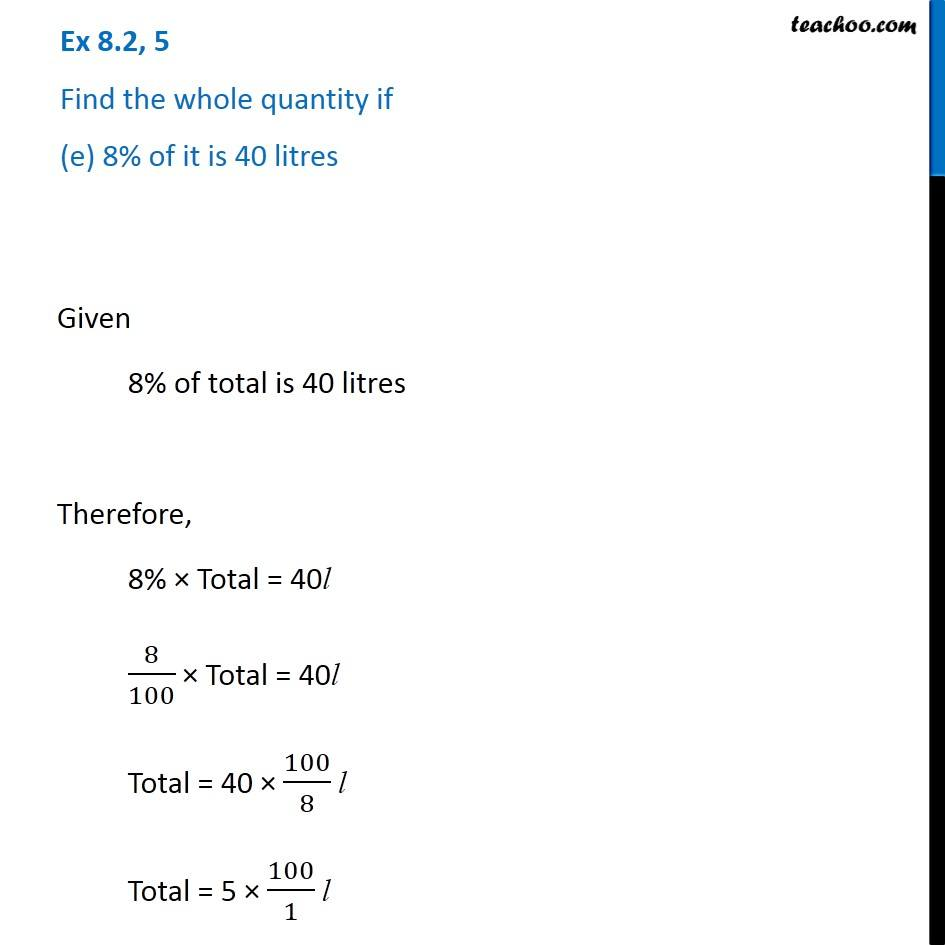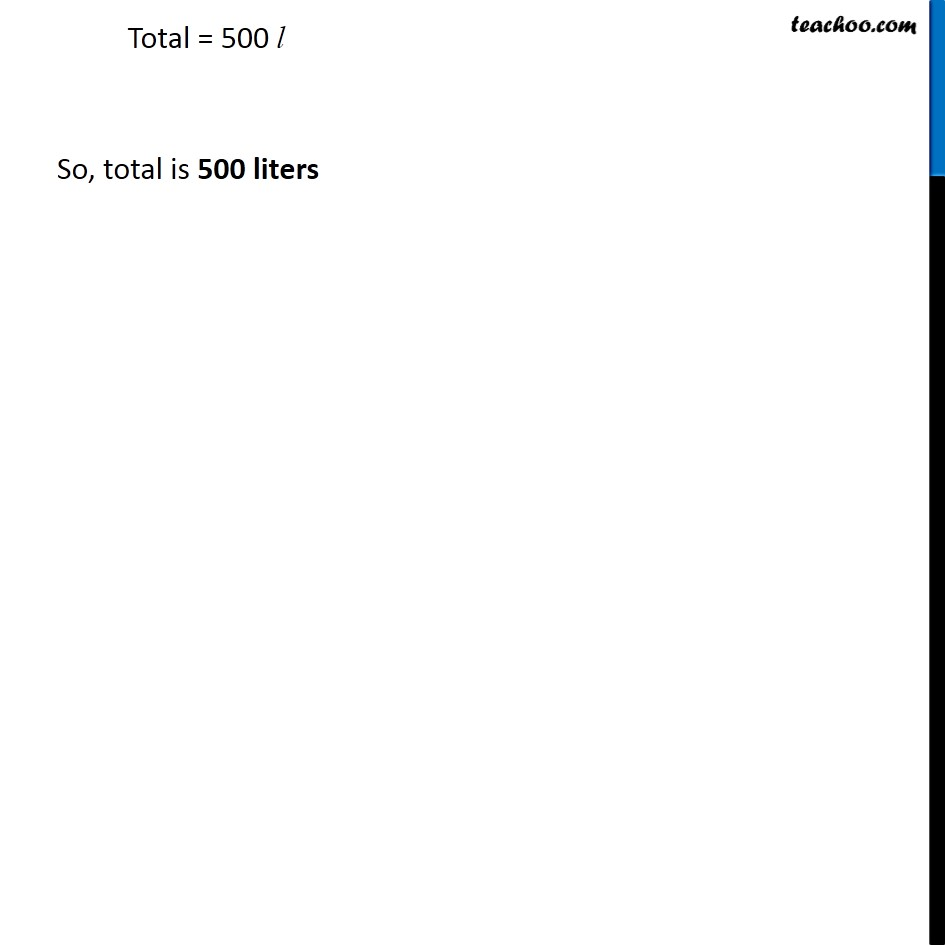1. Chapter 8 Class 7 Comparing Quantities
2. Serial order wise
3. Ex 8.2

Transcript

Ex 8.2, 5 Find the whole quantity if (e) 8% of it is 40 litres Given 8% of total is 40 litres Therefore, 8% × Total = 40l 8/100 × Total = 40l Total = 40 × 100/8 l Total = 5 × 100/1 l Total = 500 l So, total is 500 liters